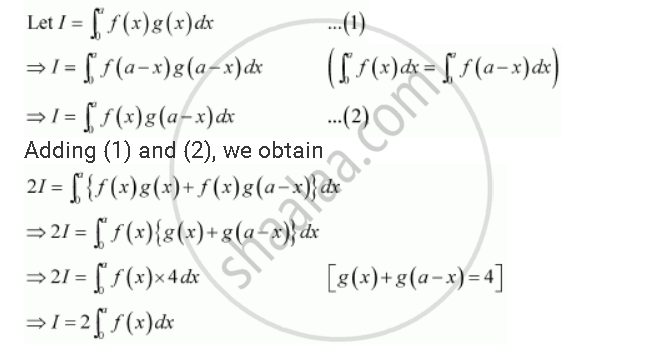Share
Notifications

View all notifications
Books Shortlist
Your shortlist is empty

# Solution for Show that Int_0^A F(X)G (X)Dx = 2 Int_0^A F(X) Dx If F And G Are Defined as F(X) = F(A-x) and G(X) + G(A-x) = 4 - CBSE (Commerce) Class 12 - Mathematics

Login
Create free account

Forgot password?
ConceptProperties of Definite Integrals

#### Question

Show that int_0^a f(x)g (x)dx = 2 int_0^a f(x) dx if f and g are defined as f(x) = f(a-x) and g(x) + g(a-x) = 4

#### SolutionIs there an error in this question or solution?

#### Video TutorialsVIEW ALL 

Solution Show that Int_0^A F(X)G (X)Dx = 2 Int_0^A F(X) Dx If F And G Are Defined as F(X) = F(A-x) and G(X) + G(A-x) = 4 Concept: Properties of Definite Integrals.
S